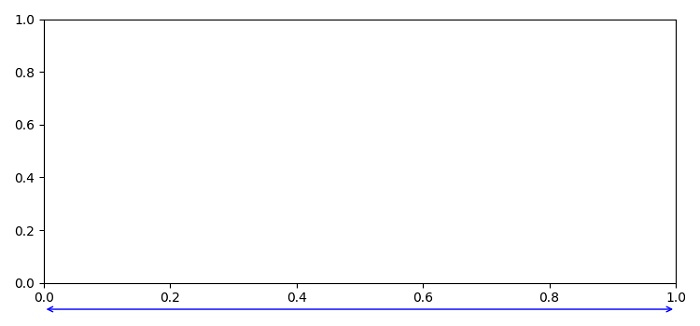# How to draw a line outside of an axis in Matplotlib?

To draw a line (i.e., arrow) outside of an axis, we can use annotate() method,

## Steps

• Set the figure size and adjust the padding between and around the subplots.

• Create a new figure or activate an existing figure using figure() method.

• Clear the current figure.

• Add an '~.axes.Axes' to the figure as part of a subplot arrangement using add_subplot() method.

• Use annotate() method to place a line outside the axes.

• To display the figure, use show() method.

## Example

import matplotlib.pyplot as plt

plt.rcParams["figure.figsize"] = [7.50, 3.50]
plt.rcParams["figure.autolayout"] = True

fig = plt.figure(1)
fig.clf()

plt.show()